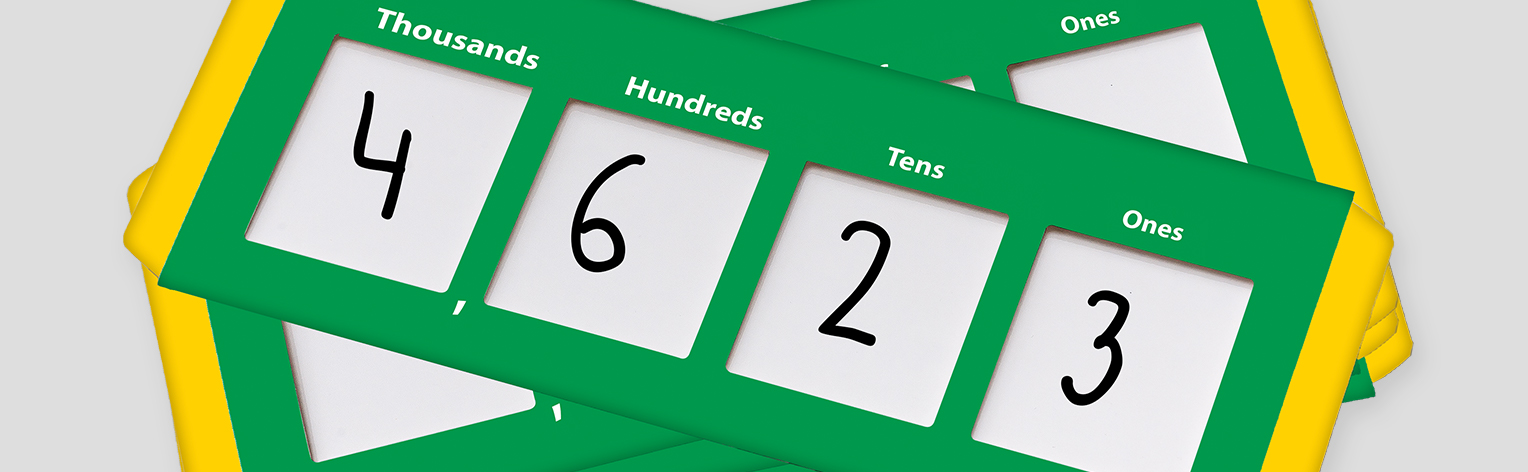When I was teaching high school, place value was a concept that just seemed to exist; it was inherent in everything I taught, yet received little attention. I simply took it for granted. As I transitioned to working with students and teachers in the elementary grades, I realized that this was a mistake because place value was a concept that many of my students probably never fully understood.

Place value is far more than just ones, tens, and hundreds. To really understand the concept of place value, we need to understand the relationship between the places. For example, we need to see that a 3 in the hundreds place has a value ten times that of a 3 in the tens place. To most of us, this is a natural conclusion, but how do we help students see this relationship?

One tool that helps students build an understanding of this concept is the place value slider. Starting with a simple number like 40, students can slide the tool to the right to see the relationship between ones and tens; sliding to the left illustrates the relationship between tens and hundreds. This tool makes it easy for students to visualize changes that are otherwise abstract.

The slider can also help students explore more complex numbers. For example, starting with the number 720, students can see what happens when dividing by 10 (sliding right) or multiplying by 10 (sliding left). A larger slider allows older students to explore numbers to millions, and simply adding a decimal point lets us explore numbers to thousandths.

Once students have an understanding of the relationships between the places, there are opportunities for group work. With students in pairs, each student can write a number on their place value slider. Then challenge students to make their number bigger or smaller than their partner’s number by sliding left or right. Students should name each number as they change them and state why their number is larger or smaller than their partner’s number. This activity can be repeated throughout the year to reinforce place value concepts.

Taking the time to allow students to explore these relationships while providing a visual model to support their learning should not be taken for granted. If we are to help students build a solid mathematical foundation, then the time students spend with place value needs to be more than numbers—it needs to be about relationships.